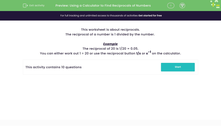# Use a Calculator to Find Reciprocals of Numbers

In this worksheet, students will practise finding reciprocals of numbers using a calculator.Key stage:  KS 3

Curriculum topic:   Number

Curriculum subtopic:   Use Calculators/Technology for Accuracy

Difficulty level:#### Worksheet Overview

What is a reciprocal?

Well, a reciprocal is a multiplicative inverse of a number.

But what does that mean?

This means that when a number is paired with its reciprocal and then multiplied together the answer will be 1

How do we find the reciprocal?

Put simply, the reciprocal of a number is 1 divided by the number.

Example

The reciprocal of 20 is 1 ÷ 20 = 1/20 or 0.05

The reciprocal of 0.2 is 1 ÷ 0.2 =  1/0.2 or 5

The reciprocal of 2/3 is 1 ÷ 2/3 = 3/2

You can either work out 1 ÷ 20 or use the reciprocal button 1/x or x-1 on the calculator.

Let's have a go at some questions.

### What is EdPlace?

We're your National Curriculum aligned online education content provider helping each child succeed in English, maths and science from year 1 to GCSE. With an EdPlace account you’ll be able to track and measure progress, helping each child achieve their best. We build confidence and attainment by personalising each child’s learning at a level that suits them.

Get started